﻿ 随机矩阵非1特征值的新包含区域 The New Inclusion Region of Eigenvalue Different from 1 for a Stochastic Matrix

Pure Mathematics
Vol.06 No.04(2016), Article ID:18153,7 pages
10.12677/PM.2016.64051

The New Inclusion Region of Eigenvalue Different from 1 for a Stochastic Matrix

Baoxing Zhou1, Huifang Wei2, Yaotang Li1*

1School of Mathematics and Statistics, Yunnan University, Kunming Yunnan

2College of Statistics and Mathematics, Yunnan University of Finance and Economics, Kunming YunnanReceived: Jul. 12th, 2016; accepted: Jul. 26th, 2016; published: Jul. 29th, 2016

Copyright © 2016 by authors and Hans Publishers Inc.ABSTRACT

Two new inclusion regions of eigenvalue different from 1 of stochastic matrices are given by using the a-eigenvalue inclusion theorem and the theory of modified matrices; and two new sufficient conditions of stochastic matrices nonsingular are obtained. Numerical examples are given to show that the existing results are improved in some cases.

Keywords:Stochastic Matrices, a1-Matrices, Eigenvalue Different from 1, a-Eigenvalue Inclusion Theorem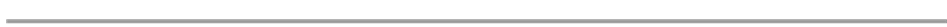1云南大学，数学与统计学院，云南 昆明

2云南财经大学，统计与数学学院，云南 昆明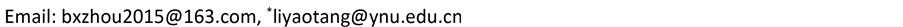1. 引言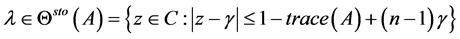Shen等在文  中通过给出随机矩阵非奇异的三个充分条件，得到了随机矩阵非1实特征值的三个包含集。随后，Li等在文  中推广了Shen的结果，得到如下定理。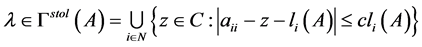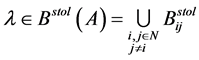2. 随机矩阵新的非1特征值包含定理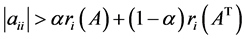,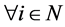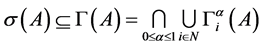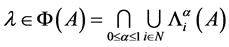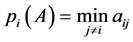,,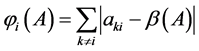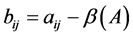,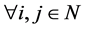(2.1)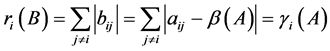(2.2)(2.3)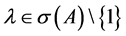，由引理2.4得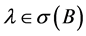，由定理2.3知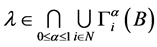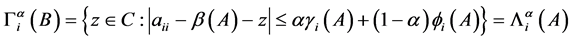,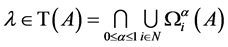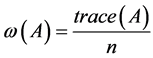,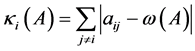,.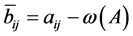,(2.4)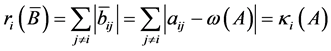(2.5)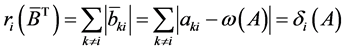(2.6),由引理2. 4得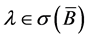,再由定理2. 3知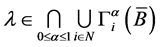,3. 随机矩阵非奇异的两个新充分条件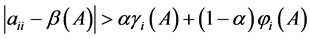,,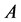是非奇异的，其中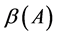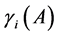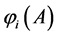同定理2.5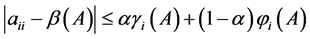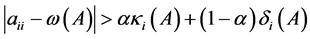,是非奇异的，其中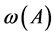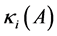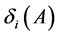同定理2.6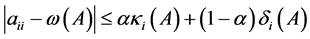4. 数值例子.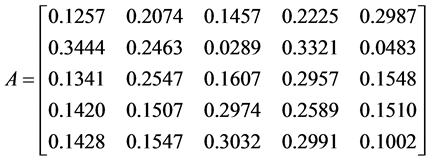..Figure 1. The comparison of Φ(A), Γstol(A), Θsto(A)

K=10; A=rand(k,k); A =inv(diag(sum(A')))*A.Figure 2. The comparison of Φ(A), Bstol(A), Θsto(A)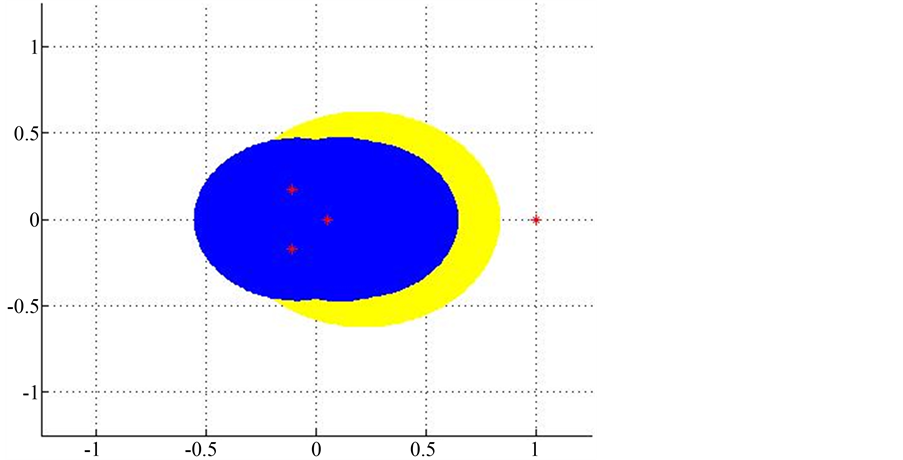Figure 3. The comparison of Γstol(A) and Φ(A)Table 2. The comparison of T(A), Γstol(A) and Bstol(A)

K=10; A =rand(k,k); A =inv(diag(sum(A')))*A.

The New Inclusion Region of Eigenvalue Different from 1 for a Stochastic Matrix[J]. 理论数学, 2016, 06(04): 361-367. http://dx.doi.org/10.12677/PM.2016.64051

1. 1. Horn, R.A. and Johnson, C.R. (1986) Matrix Analysis. Cambridge University Press, Cambridge, England.

2. 2. Seneta, E. (2004) Nonnegative Matrices and Markov Chains. Springer-Verlag, Berlin.

3. 3. Cvetković, L., Kostic, V. and Pena, J.M. (2011) Eigenvalue Localization Refinements for Matrices Related to Positivity. SIAM Journal on Matrix Analysis and Applications, 32, 771-784. http://dx.doi.org/10.1137/100807077

4. 4. Varga, R.S. (2004) Gersgorin and His Circles. Springer-Verlag, Berlin. http://dx.doi.org/10.1007/978-3-642-17798-9

5. 5. Cvetkovic, L., Kostic, V. and Varga, R.S. (2004) A New Gersgorin-Type Eigenvalue Inclusion Set. Electronic Transactions on Numerical Analysis, 18, 73-80.

6. 6. Shen, S.Q., Yu, J. and Huang, T.Z. (2014) Some Classes of Nonsingular Matrices with Applications to Localize the Real Eigenvalues of Real Matrices. Linear Algebra and Its Applications, 447, 74-87. http://dx.doi.org/10.1016/j.laa.2013.02.005

7. 7. Li, C.Q., Liu, Q.B. and Li, Y.T. (2014) Gersgorin-Type and Brauer-Type Eigenvalue Localization Sets of Stochastic Matrices. Linear and Multilinear Algebra.

8. 8. Cvetković, L. (2007) H-Matrix Theory vs. Eigenvalue Localization. Numerical Algorithms, 42, 229-245.